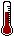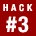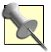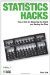# Hack 3. Figure the OddsWill I win the lottery? Will I get struck by lightning and hit by a bus on the same day? Will my basketball team have to meet our hated rival early in the NCAA tournament? At its core, statistics is all about determining the likelihood that something will happen and answering questions like these. The basic rules for calculating probability allow statisticians to predict the future.

This book is full of interesting problems that can be solved using cool statistical tricks. While all the tools presented in these hacks are applied in different ways in different contexts, many of the procedures used in these clever solutions work because of a common core set of elements: the rules of probability.

The rules are a key set of simple, established facts about how probability works and how probabilities should be calculated. Think of these two basic rules as a set of tools in a beginner's toolbox that, like a hammer and screwdriver, are probably enough to solve most problems:

The probability of any one of several independent events occurring is the sum of each event's probability.

Multiplicative rule

The probability of a series of independent events all occurring is the product of each event's probability.

These two tools will be enough to answer most of your everyday "What are the chances?" questions.

When a statistician says something like "a 1 out of 10 chance of happening," she has just made a prediction about the future. It might be a hypothetical statement about a series of events that will never be tested, or it might be an honest-to-goodness statement about what is about to happen. Either way, she's making a statistical statement about the likelihood of an outcome, which is just about all statisticians ever say [Hack #1].If the following statement makes some intuitive sense to you, then you have all the ability necessary to act and think like a stat hacker: "If there are 10 things that might happen and all 10 things are equally likely to happen, then any 1 of those things has a 1 out of 10 chance of happening."

Research is full of questions that are answered using statistics, of course, and probability rules apply, but there are many problems in the world outside the laboratory that are more important than any stupid old science problemlike games with dice, for example! Imagine you are a part-time gambler, baby needs a new pair of shoes and all that, and the values showing the next time you throw a pair of dice will determine your future. You might want to know the likelihood of various outcomes of that dice roll. You might want to know that likelihood very precisely!

You can answer the three most important types of probability questions that you are likely to ask using only your two-piece probability toolkit. Your questions probably fall into one of these three types:

• How likely is it that a specific single outcome of interest will occur next? For example, will a dice roll of 7 come up next?

• How likely is it that any of a group of outcomes of interest will occur next? For example, will either a 7 or 11 come up next?

• How likely is it that a series of outcomes will occur? For example, could an honest pair of dice really be thrown all night and a 7 never (I mean never!) come up?! I mean, really, could it?! Could it?!

## Probability Jargon

Before we talk about probability and how to determine it, we need to learn how to talk like a statistician. Remember the "1 out of 10 chance of happening"statement? Here are three ways of answering the question "What are the chances?":

As a percentage

1 out of 10 can be expressed as 10 percent.

As odds

The odds in a 1 out of 10 situation are 9 to 1 againsti.e., nine chances of losing against one chance of winning.

As a proportion

10 percent can be expressed as 0.10. Technically, probabilities should be expressed as proportions or they should be called something else.

#### Likelihood of a Specific Outcome

When you are interested in whether something is likely to happen, that "something" can be called a winning event (if you are talking about a game) or just an outcome of interest (if you are talking about something other than a game). The primary principle in probability is that you divide the number of outcomes of interest by the total number of outcomes. The total number of outcomes is sometimes symbolized with an S (for set), and all the different outcomes of interest are sometimes symbolized as A (because it is the first letter of the alphabet, I guess; what am I, a mathematician?).

So, here's the basic equation for probability:

Figuring the chances of any particular outcome or event is a matter of counting the number of those outcomes, counting the number of all possible outcomes, and comparing the two. This is easily done in most situations with a small number of possible outcomes or a description of a winning outcome that is simple and involves a single event.

To answer a typical dice roll question, we can determine the chances of any specific value showing up on the next roll by counting the number of possible combinations of two six-sided dice that adds up to the value of interest. Then, divide that number by the total number of possible outcomes. With two 6-sided dice, there are 36 possible rolls.

For example, there are six ways to throw a 7 (I peeked ahead to Table 1-2), and 6/36 = .167, so the percentage chance of throwing a 7 on any single roll is about 17 percent.Calculate the total number of possible dice rolls, or outcomes, by multiplying the total number of sides on each die: 6x6 = 36.

#### Likelihood of a Group of Outcomes

If you are interested in whether any of a group of specific outcomes will occur, but you don't care which one, the additive rule states that you can figure your total probability by adding together all the individual probabilities. To answer our dice questions, Table 1-2 borrows some information from "Play with Dice and Get Lucky" [Hack #43] to express probability for various dice rolls as proportions.

##### Table Probability of independent dice rolls
Dice rollNumber of outcomesProbability
210.028
320.056
430.083
540.111
650.139
760.167
850.139
940.111
1030.083
1120.056
1210.028
Total361.0

Table 1-2 provides information for various outcomes. For example, there are two different ways to roll a 3. Two winning outcomes divided by a total of 36 different possible outcomes results in a proportion of .056. So, about 6 percent of the time you'll roll a 3 with two dice. Notice also that the probabilities for every possible event add up to a perfect 1.0.

Let's apply the additive rule to see the chances of winning when, to win, we must get any one of several different dice rolls. If you will win with a roll of a 10, 11, or 12, for instance, add up the three individual probabilities:

.083 + .056 + .028 = .167

You will roll a 10, 11, or 12 about 17 percent of the time. The additive rule is used here because you are interested in whether any one of several independent events will happen.

#### Likelihood of a Series of Outcomes

What about when the probability question is whether more than one independent event will happen? This question is usually asked when you want to know whether a sequence of specific events will occur. The order of the events usually doesn't matter.

Using the data in Table 1-2 and the same three values of interest from our previous example (10, 11, and 12), we can figure the chance of a particular sequence of events occurring. What is the probability that, on a given series of three dice rolls in a row, you will roll a 10, an 11, and a 12? Under the multiplicative rule, multiply the three individual probabilities together:

.083x.056x.028 = .00013

This very specific outcome is very unlikely. It will happen less than .1 percent, or 1/10 of 1 percent of the time. The multiplicative rule is used here because you are interested in whether all of several independent events will happen.

#### What Probability Means

This hack talks about probability as the likelihood that something will happen. As I have placed our discussion within the context of analyzing possible outcomes, this is an appropriate way to think about probability. Among philosophers and social scientists who spend a lot of time thinking about concepts such as chance and the future and what's for lunch, there are two different views of probability.

##### Analytic view

This classic view of probability is the view of the mathematician and the approach used in this hack. The analytic view identifies all possible outcomes and produces a proportion of winning outcomes to all possible outcomes. That proportion is the probability.

We are predicting the future with the probability statement, and the accuracy of the prediction is unlikely to ever be tested. It is like when the weather forecaster says there is a 60 percent chance of rain. When it doesn't rain, we unfairly say the forecast was wrong, though, of course, we haven't really tested the accuracy of the probability statement.

##### Relative frequency view

Under the framework of this competing view, the probability of events is determined by collecting data and seeing what actually happened and how often it happened. If we rolled a pair of dice a thousand times and found that a 10 or an 11 or a 12 came up about 17 percent of the time, we would say that the chance of rolling one of those values is about 17 percent.

Our statement would really be about the past, not a prediction of the future. One might assume that past events give us a good idea of what the future holds, but who can know for sure? (Those of us who hold the analytic view of probability can know for sure, that's who.)Statistics Hacks: Tips & Tools for Measuring the World and Beating the Odds
ISBN: 0596101643
EAN: 2147483647
Year: 2004
Pages: 114
Authors: Bruce Frey

Similar book on Amazon